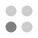• 5,004 trophies earned
• 247 Players Tracked
• 21 Total trophies
• 210 Obtainable EXP
• 1,170 Points
• 232 Platinum Club
• 232 100% Club

### Energy Cycle Edge Trophies

•94.74%
•Solved first puzzle100.00%
•Puzzles 2, 3 and 4 solved97.98%
•Puzzles 5, 6 and 7 solved97.57%
•Puzzles 8, 9 and 10 solved97.57%
•Puzzles 11, 12 and 13 solved97.17%
•Puzzles 14, 15 and 16 solved96.36%
•Puzzles 17, 18 and 19 solved95.95%
•Puzzles 20, 21 and 22 solved95.95%
•Puzzles 23, 24 and 25 solved95.95%
•Puzzles 26, 27 and 28 solved95.55%
•Puzzles 29, 30 and 31 solved95.55%
•Puzzles 32, 33 and 34 solved95.55%
•Puzzles 35 and 36 solved95.55%
•Puzzles 37, 38 and 39 solved95.95%
•Puzzles 40 and 41 solved95.55%
•Puzzles 42 and 43 solved95.55%
•All of puzzles solved95.14%
•Took less than 10 seconds to solve the puzzle96.76%
•Took more than 15 minutes to solve the puzzle96.76%
•3D puzzle is solved exactly with one rotation97.57%